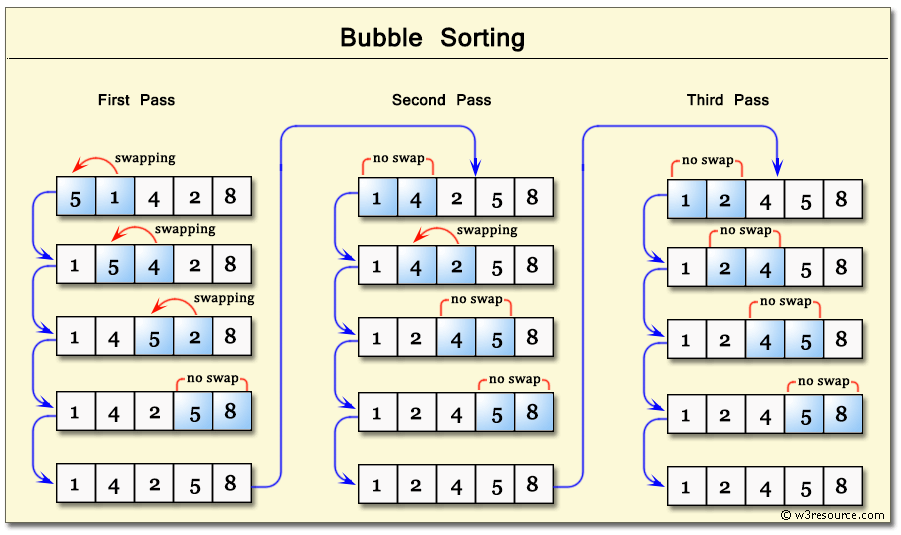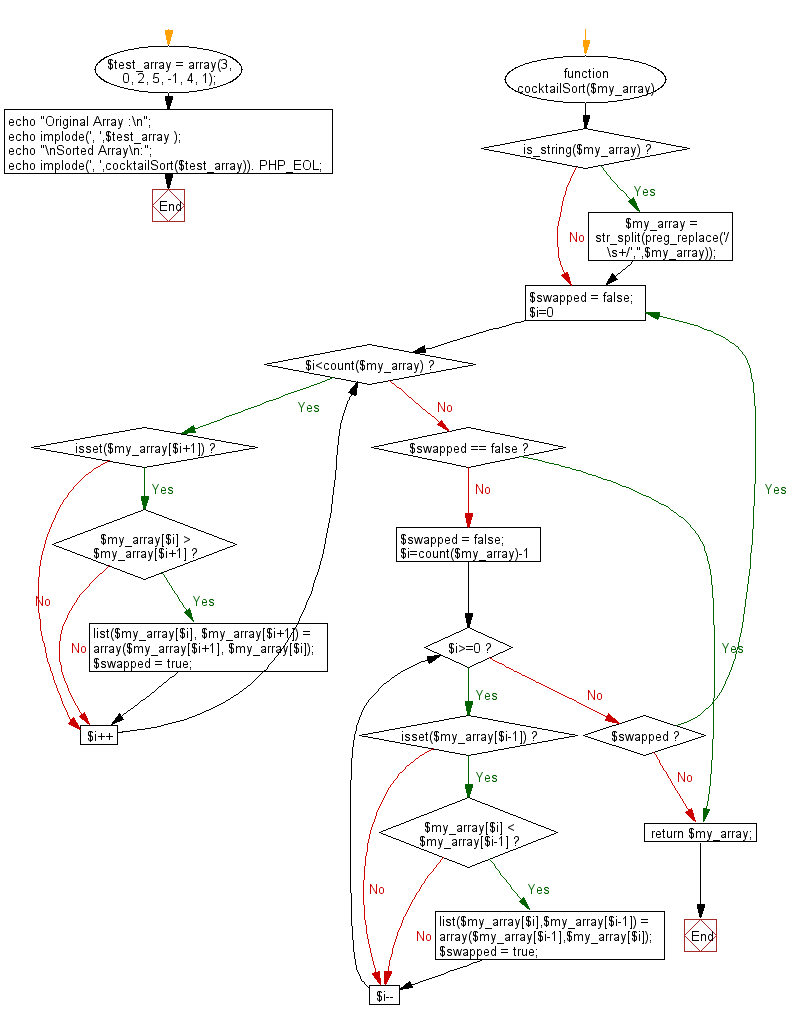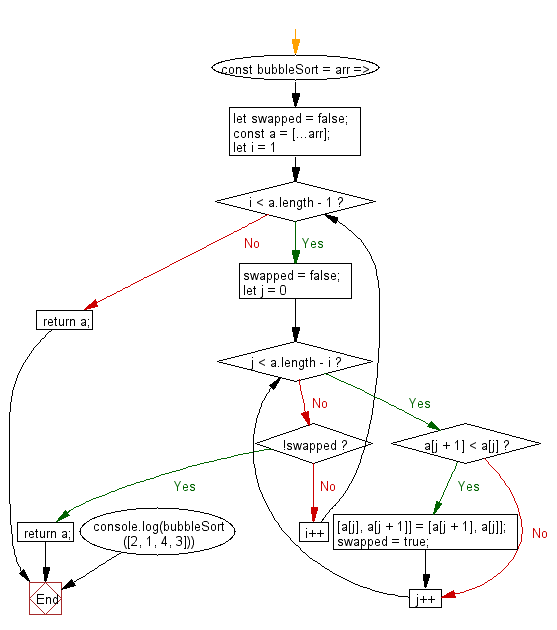# JavaScript Sorting Algorithm: Sorts an array of numbers, using the bubble sort algorithm

## JavaScript Sorting Algorithm: Exercise-7 with Solution

Write a JavaScript program to sort a list of elements using Bubble sort.

According to Wikipedia "Bubble sort, sometimes referred to as sinking sort, is a simple sorting algorithm that repeatedly steps through the list to be sorted, compares each pair of adjacent items and swaps them if they are in the wrong order. The pass through the list is repeated until no swaps are needed, which indicates that the list is sorted. The algorithm, which is a comparison sort, is named for the way smaller elements "bubble" to the top of the list. Although the algorithm is simple, it is too slow and impractical for most problems even when compared to insertion sort. It can be practical if the input is usually in sort order but may occasionally have some out-of-order elements nearly in position."

Step by step pictorial presentation :Sample Solution-1:

JavaScript Code:

``````function swap(arr, first_Index, second_Index){
var temp = arr[first_Index];
arr[first_Index] = arr[second_Index];
arr[second_Index] = temp;
}

function bubble_Sort(arr){

var len = arr.length,
i, j, stop;

for (i=0; i < len; i++){
for (j=0, stop=len-i; j < stop; j++){
if (arr[j] > arr[j+1]){
swap(arr, j, j+1);
}
}
}

return arr;
}
console.log(bubble_Sort([3, 0, 2, 5, -1, 4, 1]));
```
```

Sample Output:

```[-1,0,1,2,3,4,5]
```

Flowchart:Sample Solution-2:

• Declare a variable, swapped, that indicates if any values were swapped during the current iteration.
• Use the spread operator (...) to clone the original array, arr.
• Use a for loop to iterate over the elements of the cloned array, terminating before the last element.
• Use a nested for loop to iterate over the segment of the array between 0 and i, swapping any adjacent out of order elements and setting swapped to true.
• If swapped is false after an iteration, no more changes are needed, so the cloned array is returned.

JavaScript Code:

``````const bubbleSort = arr => {
let swapped = false;
const a = [...arr];
for (let i = 1; i < a.length - 1; i++) {
swapped = false;
for (let j = 0; j < a.length - i; j++) {
if (a[j + 1] < a[j]) {
[a[j], a[j + 1]] = [a[j + 1], a[j]];
swapped = true;
}
}
if (!swapped) return a;
}
return a;
};

console.log(bubbleSort([2, 1, 4, 3]))
```
```

Sample Output:

```[1,2,3,4]
```

Flowchart:Live Demo:

See the Pen searching-and-sorting-algorithm-exercise-7 by w3resource (@w3resource) on CodePen.

Improve this sample solution and post your code through Disqus

What is the difficulty level of this exercise?

Test your Programming skills with w3resource's quiz.

﻿